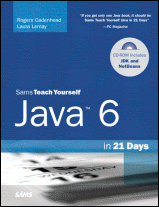# Java 6 in 21 Days (5th Edition) Day 5: Creating Classes and Methods## Notes and Corrections

• View the Certification Practice quiz solution
• Certification answer: Answer (c) is correct. The constructor method of the BiggerValue class calls the method calculateResult(int, int, int). Because this method is not defined in the BigValue class, it must be defined in the BiggerValue class.

Answers (a) is incorrect. It would produce a result equal to 312.0 if the constructor method of the BiggerValue class called calculateResult(int) with the c variable as an argument, but the constructor calls calculateResult(int, int, int).

Answers (b) and (d) are incorrect. Using either of these statements, the example will not compile because the constructor method of the BiggerValue class uses a variable called c which is not defined in the constructor.

• Page 141: The first answer in the Q&A should state that the easiest way to avoid a variable scope problem is not to give your local variables the same names as your instance variables.